Skip to content
Related Articles
Python | Corner Detection with Shi-Tomasi Corner Detection Method using OpenCV
• Last Updated : 22 Aug, 2019

What is a Corner?
A corner can be interpreted as the junction of two edges (where an edge is a sudden change in image brightness).

### Shi-Tomasi Corner Detection –

Shi-Tomasi Corner Detection was published by J.Shi and C.Tomasi in their paper ‘Good Features to Track‘. Here the basic intuition is that corners can be detected by looking for significant change in all direction.

We consider a small window on the image then scan the whole image, looking for corners.

Shifting this small window in any direction would result in a large change in appearance, if that particular window happens to be located on a corner.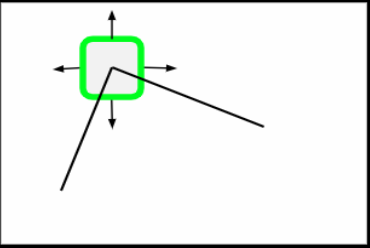Flat regions will have no change in any direction.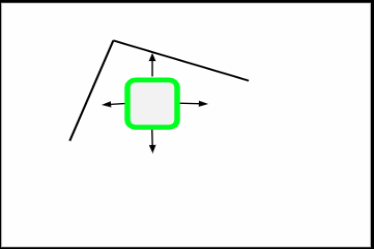If there’s an edge, then there will be no major change along the edge direction.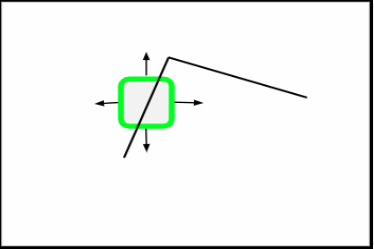### Mathematical Overview –

For a window(W) located at (X, Y) with pixel intensity I(X, Y), formula for Shi-Tomasi Corner Detection is –

`f(X, Y) = Σ (I(Xk, Yk) - I(Xk + ΔX, Yk + ΔY))2  where (Xk, Yk) ϵ W`

According to the formula:
If we’re scanning the image with a window just as we would with a kernel and we notice that there is an area where there’s a major change no matter in what direction we actually scan, then we have a good intuition that there’s probably a corner there.

Calculation of f(X, Y) will be really slow. Hence, we use Taylor expansion to simplify the scoring function, R.

```R = min(λ1, λ2)
where λ1, λ2 are eigenvalues of resultant matrix```

Using `goodFeaturesToTrack()` function –

Syntax : cv2.goodFeaturesToTrack(gray_img, maxc, Q, minD)

Parameters :
gray_img – Grayscale image with integral values
maxc – Maximum number of corners we want(give negative value to get all the corners)
Q – Quality level parameter(preferred value=0.01)
maxD – Maximum distance(preferred value=10)

Below is the Python implementation of Shi-Tomasi Corner Detection:
 `# Python program to illustrate ``# corner detection with ``# Shi-Tomasi Detection Method``   ` `# organizing imports ``import` `cv2``import` `numpy as np``import` `matplotlib.pyplot as plt``%``matplotlib inline`` ` `# path to input image specified and  ``# image is loaded with imread command``img ``=` `cv2.imread(``'chess.png'``)`` ` `# convert image to grayscale``gray_img ``=` `cv2.cvtColor(img, cv2.COLOR_BGR2GRAY)`` ` `# Shi-Tomasi corner detection function``# We are detecting only 100 best corners here``# You can change the number to get desired result.``corners ``=` `cv2.goodFeaturesToTrack(gray_img, ``100``, ``0.01``, ``10``)`` ` `# convert corners values to integer``# So that we will be able to draw circles on them``corners ``=` `np.int0(corners)`` ` `# draw red color circles on all corners``for` `i ``in` `corners:``    ``x, y ``=` `i.ravel()``    ``cv2.circle(img, (x, y), ``3``, (``255``, ``0``, ``0``), ``-``1``)`` ` `# resulting image``plt.imshow(img)`` ` `# De-allocate any associated memory usage  ``if` `cv2.waitKey(``0``) & ``0xff` `=``=` `27``: ``    ``cv2.destroyAllWindows()`

Input :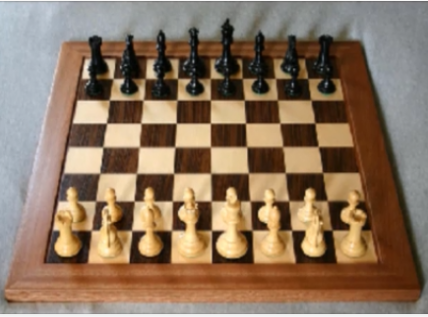Output :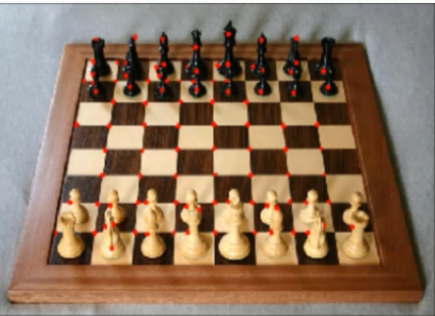Attention geek! Strengthen your foundations with the Python Programming Foundation Course and learn the basics.

To begin with, your interview preparations Enhance your Data Structures concepts with the Python DS Course. And to begin with your Machine Learning Journey, join the Machine Learning – Basic Level Course

My Personal Notes arrow_drop_up